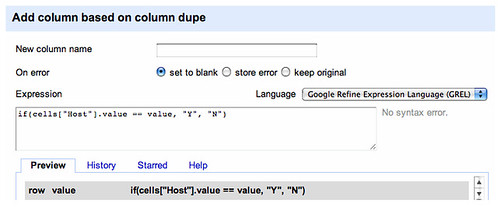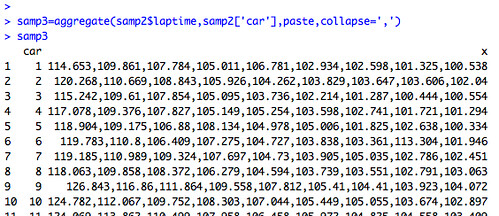# Comparing Columns in Google Refine

A reader (Cosmin Cabulea) writes: “I have two columns (A and B) and want to identify identical cells.”

I think I misapprehended the point of the question, but it prompted me to create this simple example.

In something like Google Spreadsheets, we could use an if statement to set the value of cells in a new column based on a comparison of the values of two other columns in the same row. In column C, cell C1, for example, we might use a formula of the form:

if(A1=B1,'similar','different')

In Google Refine, we can use a GREL expression to achieve a similar effect. Create a new column and then use an expression of the form:

if(cells["A"].value == cells["B"].value, "similar", "different")

where A and B are the appropriate column headings.If you’re generating the new comparison column from one of the two columns you’re comparing (column with header B, say), you can reference the values of the original column directly:

if(cells["A"].value == value, "similar", "different")It strikes me that the pattern scales to comparisons across multiple columns and of arbitrary complexity. For example, using a nested if control flow statement:

if( value == cells["Host"].value, if( cells["amount"].value > 75, 2, 1 ), 0 )

Or using a Boolean operator:

if( and( value=="May",cells['amount'].value > 0 ), 2, 0 )

Aggregating Values for Recurring Column Values

So this, it turns out (I think?!), was more in line with what Cosmin was after. Given something like:

A B
1 2
1 3
2 1
2 3
2 4

generate:

A BVALS
1 2,3
2 2,3,4

Here’s a way of doing that using R (I use the R-Studio environment).

Using some (guess what) F1 data, loaded into the dataframe hun_2011proximity, let’s pull out a sample of laptime data (say the first 10 laps of a race), featuring just the car numbers, and the laptimes (ref: R: subsetting data). First we grab just those rows where the lap column value is less than 11, then we create a frame containing only a couple of the columns (car and laptime) from the dataset (the original hun_2011proximity data frame contained 20 or so columns, including two with headers car and laptime, and 70 laps worth of data):

samp1=subset(hun_2011proximity,lap<11)
sampcols=c("car","laptime")
samp2=samp1[sampcols]

(Thinks: would it be more efficient to do this the other way round, and reduce the data set to 2 cols first before extracting just the first 10 laps worth of data?)

samp2 now contains 240 rows describing 10 laps of data, each row containing data for one car from one lap; each row contains car and laptime data (2 cols).

Now we can run down one column, looking for recurring elements, and generate a new column that contains the aggregate values from another column for each unique element in the first column:

samp3=aggregate(samp2\$laptime, samp2['car'],paste,collapse=',')

Here’s what we get as a result:A Couple of Alternative Approaches
Chatting to Cosmin, it turns out the actual requirement was to identify common followers of a set of Twitter accounts. So for example, with columns TwitterID FollowedBy, extract the unique FollowedBy Twitter IDs and then aggregate the TwitterID values (something like aggdata=aggregate(twData\$TwitterID, twData[‘FollowedBy’],paste,collapse=’,’)).

One approach to this would be to look at the data in Gephi, plotting edges as a directed graph from FollowedBy to TwitterID, sizing the nodes according to out degree (so we could see how many of the target accounts each person in the union follower set was following). We could then use filters to reduce the set to just people following lots of the accounts.

Following this line of thought, we could also use a network flavoured representation (e.g. using something like networkx) to construct a graph and run stats on it. (So we could e.g. pull out reports describing the distribution of how many people were following how many of the target accounts, etc.)

Of course, on those occasions where the Google Social API returns Twitter follower names rather than redirect IDs, my Common Friends or Followers on Twitter hack will show common followers of two twitter accounts.

Yet another approach, if we have all the data in a single file, is to do a simple bit of counting using a Unix command line tool. For example, if we have comma separated file containing TwitterID (column 1) and FollowedBy (column 2) columns, we can sort the names in the FollowedBy column and count the number of times they reoccur:

cut -d "," -f 2 twitterdata.csv | sort | uniq -c

## Author: Tony Hirst

I'm a Senior Lecturer at The Open University, with an interest in #opendata policy and practice, as well as general web tinkering...